### ezmisc

ctfwiki中也有很详细的介绍：https://ctf-wiki.github.io/ctf-wiki/misc/picture/png-zh/

### 你能看懂音符吗

word隐写方式（供参考）：https://blog.csdn.net/q_l_s/article/details/53813971

flag：`MRCTF{thEse_n0tes_ArE_am@zing~}`

### Hello，misc_world！

• 第一种解法（其实是俺的预期解，但是师傅们给了更简单的解法，可见解法二）

``````from PIL import Image
import bitstring

with open('1.png','rb') as f:
bitcon = bitstring.Bits(f)
im = Image.new('RGB',(1024,780),(255,0,0))
for i,val in enumerate(bitcon.bin):
if val == '0':
pim[i%1024,i/1024] = (0,0,255)
im.save('red_blue.png')``````

``````from PIL import Image
import bitstring

im = Image.open('try to restore it.png')
width = im.size
height = im.size
bin_result = ''
for h in range(height):
for w in range(width):
if pim[w,h] == 255: # 判断是否是红色（R,G,B）表示第一通道
bin_result += '1'
else:
bin_result += '0'

# print bitstring.BitArray(bin=bin_result).bytes.encode('hex')
with open('result.png','wb') as f:
f.write(bitstring.BitArray(bin=bin_result).bytes)``````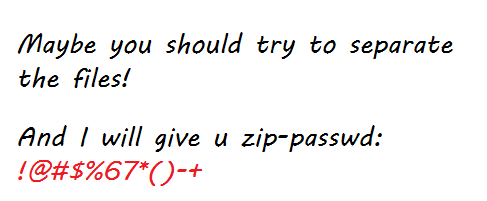• 第二种解法

`stegsolve`查看图片，`Save Bin`可直接得到图片：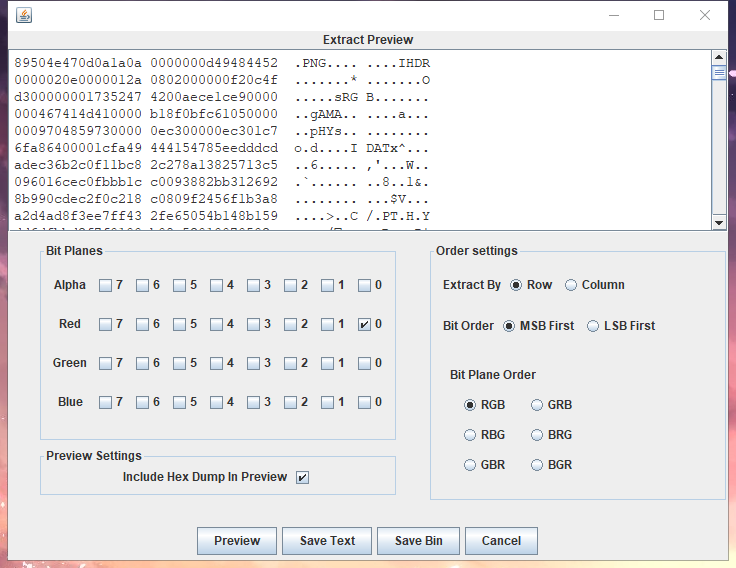``````127
255
63
191
127
191
63
127
127
255
63
191
63
191
255
127
...``````

``````fp = open('out.txt','r')
p = []
for i in a:
p.append(int(i))

s = ''
for i in p:
if i == 63:
a = '00'
elif i == 127:
a = '01'
elif i == 191:
a = '10'
elif i == 255:
a = '11'
s += a

import binascii

result = ''
for i in range(0,len(s),8):
result += chr(int(s[i:i+8],2))

print result``````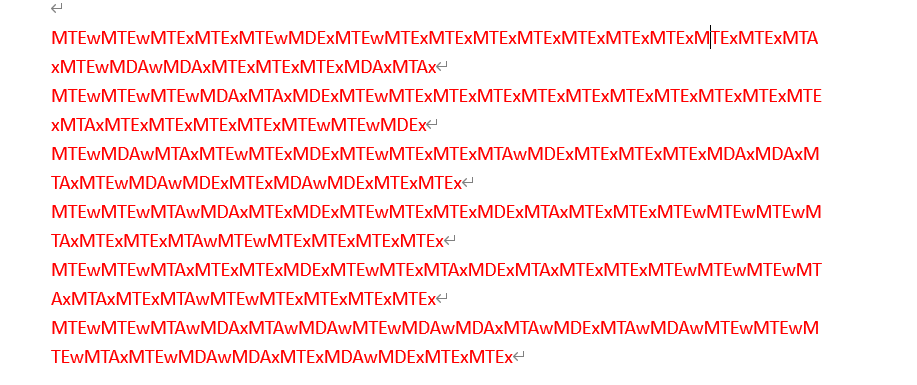ps：如果用word打开的话可以看到有最后的换行符存在，可以判断文档中隐藏了内容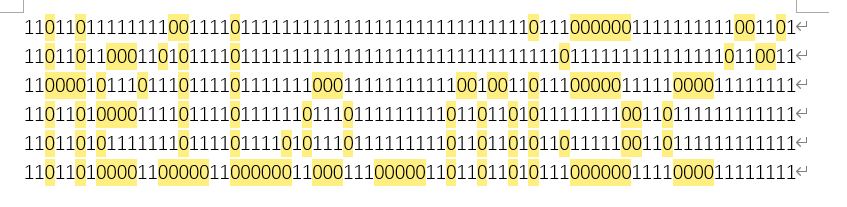``flag: MRCTF{He1Lo_mi5c~}``

### 千层套路

``````#coding=utf-8
import os
import zipfile

orginal_zip = "0573.zip"

while True:
tag = orginal_zip
orginal_zip = zipfile.ZipFile(orginal_zip)
for contents in orginal_zip.namelist():
try:
orginal_zip.extractall()
except:
break
if(len(tag)>6):
os.system("rm "+tag)

``````#!/usr/bin/env bash

while [ -e *.zip ]; do
files=*.zip;
for file in \$files; do
echo -n "Cracking \${file}… ";
if [ -z "\${password}" ]; then
break 2;
fi;
rm "\${file}";
done;
done;``````

``````(255, 255, 255)
(255, 255, 255)
(255, 255, 255)
(255, 255, 255)
(255, 255, 255)
(255, 255, 255)
(255, 255, 255)
...``````

``````#coding=utf-8
from PIL import Image

x = 200    #x坐标  通过对txt里的行数进行整数分
y = 200    #y坐标  x * y = 行数

im = Image.new("RGB", (x, y))
file = open('qr.txt')

for i in range(0, x):
for j in range(0, y):
line = line[:-2]
line = line[1:]
print line
rgb = line.split(", ")  #分离rgb，文本中逗号后面有空格
im.putpixel((i, j), (int(rgb), int(rgb), int(rgb)))

im.save('flag.png')``````

``flag：MRCTF{ta01uyout1nreet1n0usandtimes}``

### 不眠之夜

``montage *jpg -tile 10x12 -geometry 200x100+0+0 out.jpg``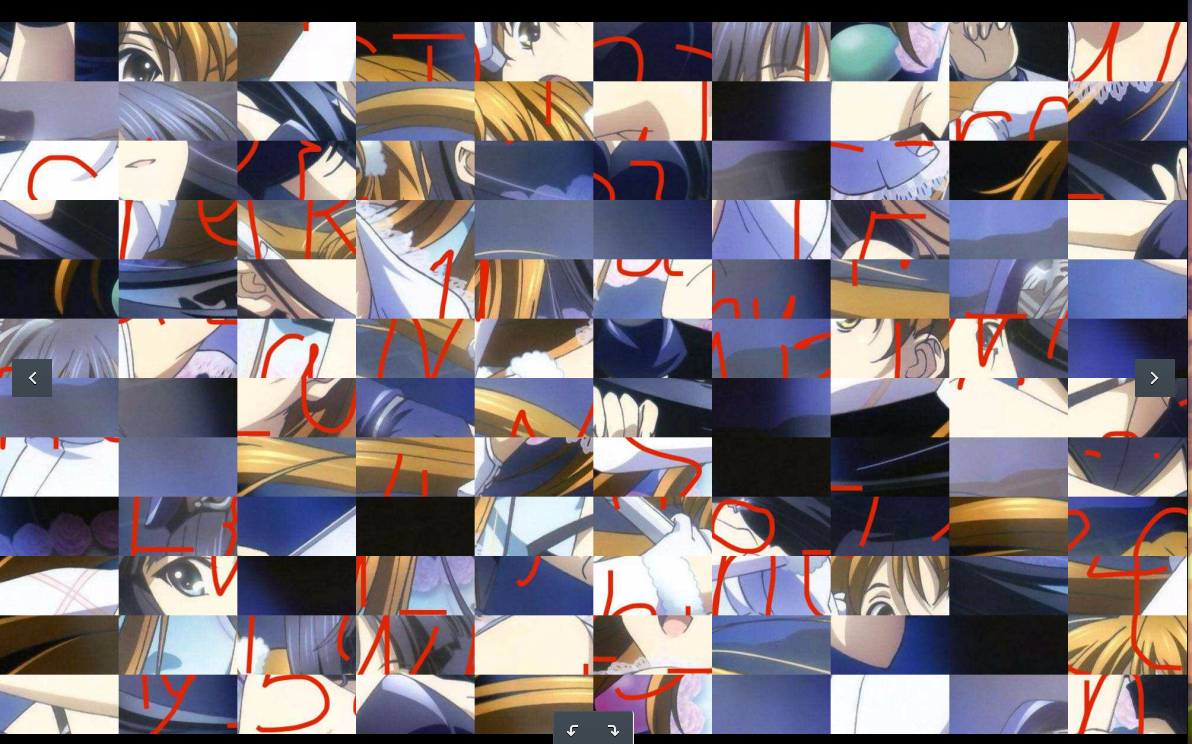``gaps --image=out.jpg --generations=40 --population=120 --size=100``### A Signal From ISS

``You received some radio voice. Warn: DO NOT PLAY IT LOUD``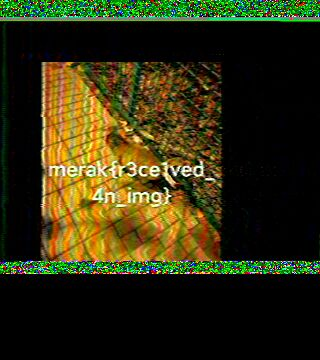### CyberPunk

flag：`MRCTF{We1cOm3_70_cyber_security}`

### Unravel!!

``U2FsdGVkX1/nSQN+hoHL8OwV9iJB/mSdKk5dmusulz4=``